JMSLTM Numerical Library 7.2.0
com.imsl.math

## Class ComplexLU

• All Implemented Interfaces:
Serializable, Cloneable

```public class ComplexLU
extends Object
implements Serializable, Cloneable```
LU factorization of a matrix of type `Complex`.

`ComplexLU` performs an LU factorization of a complex general coefficient matrix. `ComplexLU`'s method `condition` estimates the reciprocal of the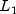condition number of the matrix. The LU factorization is done using scaled partial pivoting. Scaled partial pivoting differs from partial pivoting in that the pivoting strategy is the same as if each row were scaled to have the same infinity norm.

Thecondition number of the matrix A is defined to be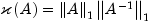. Since it is expensive to compute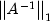, the condition number is only estimated. The estimation algorithm is the same as used by LINPACK and is described by Cline et al. (1979).

Note that A is not retained for use by other methods of this class, only the factorization of A is retained. Thus, A is a required parameter to the `condition` method.

An estimated condition number greater than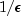(whereis machine precision) indicates that very small changes in A can cause very large changes in the solution x. Iterative refinement can sometimes find the solution to such a system. If there is conern about the input matrix being ill-conditioned, the user of this class should check the condition number of the input matrix using the `condition` method before using one of the other class methods.

`ComplexLU` fails if U, the upper triangular part of the factorization, has a zero diagonal element. This can occur only if A either is singular or is very close to a singular matrix.

The `solve` method can be used to solve systems of equations. The method `determinant` can be called to compute the determinant of the coefficient matrix.

ComplexLU is based on the LINPACK routine `CGECO`; see Dongarra et al. (1979). `CGECO` uses unscaled partial pivoting.

Example, Serialized Form
• ### Field Summary

Fields
Modifier and Type Field and Description
`protected Complex[][]` `factor`
This is an n by n `Complex` matrix containing the LU factorization of the matrix A.
`protected int[]` `ipvt`
Vector of length n containing the pivot sequence for the factorization.
• ### Constructor Summary

Constructors
Constructor and Description
`ComplexLU(Complex[][] a)`
Creates the LU factorization of a square matrix of type `Complex`.
• ### Method Summary

Methods
Modifier and Type Method and Description
`double` `condition(Complex[][] a)`
Return an estimate of the reciprocal of thecondition number.
`Complex` `determinant()`
Return the determinant of the matrix used to construct this instance.
`Complex[][]` `getL()`
Returns the lower triangular portion of the LU factorization of A.
`Complex[][]` `getPermutationMatrix()`
Returns the permutation matrix which results from the LU factorization of A.
`Complex[][]` `getU()`
Returns the unit upper triangular portion of the LU factorization of A.
`Complex[][]` `inverse()`
Returns the inverse of the matrix used to construct this instance.
`Complex[]` `solve(Complex[] b)`
Return the solution x of the linear system Ax = b using the LU factorization of A.
`static Complex[]` ```solve(Complex[][] a, Complex[] b)```
Solve Ax = b for x using the LU factorization of A.
`Complex[]` `solveTranspose(Complex[] b)`
Return the solution x of the linear system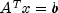.
• ### Methods inherited from class java.lang.Object

`clone, equals, finalize, getClass, hashCode, notify, notifyAll, toString, wait, wait, wait`
• ### Field Detail

• #### factor

`protected Complex[][] factor`
This is an n by n `Complex` matrix containing the LU factorization of the matrix A.
• #### ipvt

`protected int[] ipvt`
Vector of length n containing the pivot sequence for the factorization.
• ### Constructor Detail

• #### ComplexLU

```public ComplexLU(Complex[][] a)
throws SingularMatrixException```
Creates the LU factorization of a square matrix of type `Complex`.
Parameters:
`a` - `Complex` square matrix to be factored
Throws:
`IllegalArgumentException` - is thrown when the row lengths of input matrix are not equal (for example, the matrix edges are "jagged".)
`SingularMatrixException` - is thrown when the input matrix is singular.
• ### Method Detail

• #### condition

`public double condition(Complex[][] a)`
Return an estimate of the reciprocal of thecondition number.
Parameters:
`a` - a `Complex` matrix
Returns:
a `double` scalar value representing the estimate of the reciprocal of thecondition number of the matrix A, where A is the parameter `a`
• #### determinant

`public Complex determinant()`
Return the determinant of the matrix used to construct this instance.
Returns:
a `Complex` scalar containing the determinant of the matrix used to construct this instance
• #### getL

`public Complex[][] getL()`
Returns the lower triangular portion of the LU factorization of A.

Scaled partial pivoting is used to achieve the LU factorization. The resulting factorization is such that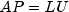, where A is the input matrix `a`, P is the permutation matrix returned by `getPermutationMatrix`, L is the lower triangular matrix returned by `getL`, and U is the unit upper triangular matrix returned by `getU`.

Returns:
a `Complex` matrix containing L, the lower triangular portion of the LU factorization of A.
• #### getPermutationMatrix

`public Complex[][] getPermutationMatrix()`
Returns the permutation matrix which results from the LU factorization of A.

Scaled partial pivoting is used to achieve the LU factorization. The resulting factorization is such that, where A is the input matrix `a`, P is the permutation matrix returned by `getPermutationMatrix`, L is the lower triangular matrix returned by `getL`, and U is the unit upper triangular matrix returned by `getU`.

Returns:
A `Complex` matrix containing the permuted identity matrix as a result of the LU factorization of A.
• #### getU

`public Complex[][] getU()`
Returns the unit upper triangular portion of the LU factorization of A.

Scaled partial pivoting is used to achieve the LU factorization. The resulting factorization is such that, where A is the input matrix `a`, P is the permutation matrix returned by `getPermutationMatrix`, L is the lower triangular matrix returned by `getL`, and U is the unit upper triangular matrix returned by `getU`.

Returns:
a `Complex` matrix containing U, the unit upper triangular portion of the LU factorization of A.
• #### inverse

`public Complex[][] inverse()`
Returns the inverse of the matrix used to construct this instance.
Returns:
a `Complex` matrix containing the inverse of the matrix used to construct this object.
• #### solve

`public Complex[] solve(Complex[] b)`
Return the solution x of the linear system Ax = b using the LU factorization of A.
Parameters:
`b` - `Complex` array containing the right-hand side of the linear system
Returns:
`Complex` array containing the solution to the linear system of equations
• #### solve

```public static Complex[] solve(Complex[][] a,
Complex[] b)
throws SingularMatrixException```
Solve Ax = b for x using the LU factorization of A.
Parameters:
`a` - a `Complex` square matrix
`b` - a `Complex` column vector
Returns:
a `Complex` column vector containing the solution to the linear system of equations.
Throws:
`IllegalArgumentException` - This exception is thrown when (1) the lengths of the rows of the input matrix are not uniform, and (2) the number of rows in the input matrix is not equal to the number of elements in x.
`SingularMatrixException` - is thrown when the matrix is singular.
• #### solveTranspose

`public Complex[] solveTranspose(Complex[] b)`
Return the solution x of the linear system.
Parameters:
`b` - `Complex` array containing the right-hand side of the linear system
Returns:
`Complex` array containing the solution to the linear system of equations
JMSLTM Numerical Library 7.2.0